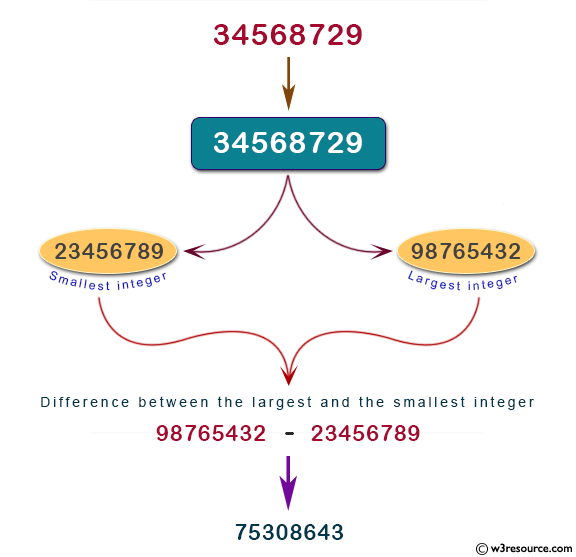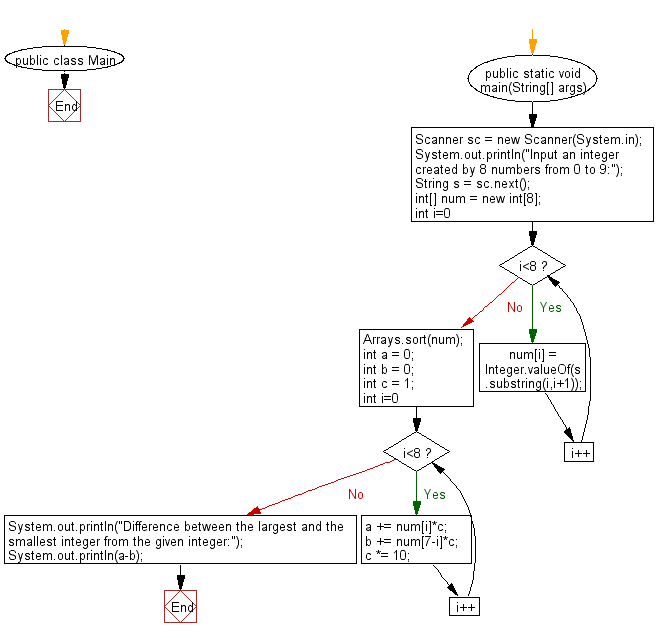# Java Exercises: Find the difference between the largest integer and the smallest integer which are created by 8 numbers from 0 to 9

## Java Basic: Exercise-231 with Solution

Write a Java program to find the difference between the largest integer and the smallest integer which are created by 8 numbers from 0 to 9. The number that can be rearranged shall start with 0 as in 00135668.

Input:

Data is a sequence of 8 numbers (numbers from 0 to 9).
Output:
The difference between the largest integer and the smallest integer.

Pictorial Presentation:Sample Solution:

Java Code:

`````` import java.util.*;
public class Main {
public static void main(String[] args) {
Scanner sc = new Scanner(System.in);
System.out.println("Input an integer created by 8 numbers from 0 to 9:");
String s = sc.next();
int[] num = new int;
for(int i=0;i<8;i++){
num[i] = Integer.valueOf(s.substring(i,i+1));
}
Arrays.sort(num);
int a = 0;
int b = 0;
int c = 1;
for(int i=0;i<8;i++){
a += num[i]*c;
b += num[7-i]*c;
c *= 10;
}
System.out.println("Difference between the largest and the smallest integer from the given integer:");
System.out.println(a-b);
}
}
```
```

Sample Output:

```Input an integer created by 8 numbers from 0 to 9:
567894321
Difference between the largest and the smallest integer from the given integer:
75308643
```

Flowchart:Java Code Editor:

What is the difficulty level of this exercise?

﻿

## Java: Tips of the Day

Parsing dates:

```import java.io.*;
import java.util.*;
import java.text.*;

String s = "2001/09/23 14:39";

SimpleDateFormat formatter = new SimpleDateFormat ("yyyy/MM/dd H:mm");
Date d = formatter.parse(s, new ParsePosition(0));
```